International
Tables for
Crystallography
Volume D
Physical properties of crystals
Edited by A. Authier

International Tables for Crystallography (2006). Vol. D, ch. 1.1, p. 16

## Section 1.1.4.6.3. The method of direct inspection

A. Authiera*

aInstitut de Minéralogie et de la Physique des Milieux Condensés, Bâtiment 7, 140 rue de Lourmel, 75015 Paris, France
Correspondence e-mail: aauthier@wanadoo.fr

#### 1.1.4.6.3. The method of direct inspection

| top | pdf |

The method of direct inspection', due to Fumi (1952a,b, 1987), is very simple. It is based on the fundamental properties of tensors; the components transform under a change of basis like a product of vector components (Section 1.1.3.2).

#### Examples

 (1) Let us consider a tensor of rank 3 invariant with respect to a twofold axis parallel to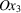. The matrix representing this operator is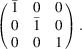The component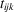behaves under a change of axes like the product of the components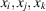. The componentsof a vector become, respectively,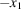,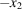,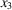. To simplify the notation, we shall denote the components of the tensor simply by ijk. If, amongst the indices i, j and k, there is an even number (including the number zero) of indices that are equal to 3, the product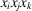will become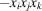under the rotation. As the component ijk' remains invariant and is also equal to its opposite, it must be zero. 14 components will thus be equal to zero: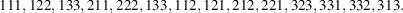(2) Let us now consider that the same tensor of rank 3 is invariant with respect to a fourfold axis parallel to. The matrix representing this operator and its action on a vector of coordinatesis given by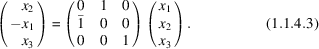Coordinate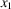becomes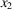,becomesandbecomes. Component ijk transforms like product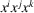according to the rule given above. Since the twofold axis parallel tois a subgroup of the fourfold axis, we can start from the corresponding reduction. We find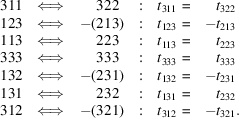All the other components are equal to zero.

It is not possible to apply the method of direct inspection for point group 3. One must in this case use the matrix method described in Section 1.1.4.6.2; once this result is assumed, the method can be applied to all other point groups.

### References

Fumi, F. G. (1952a). Physical properties of crystals: the direct inspection method. Acta Cryst. 5, 44–48.
Fumi, F. G. (1952b). The direct-inspection method in systems with a principal axis of symmetry. Acta Cryst. 5, 691–694.
Fumi, F. G. (1987). Tables for the third-order elastic tensors in crystals. Acta Cryst. A43, 587–588.